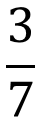1
visibility

There were 7 pupils in a classroom. 4 went out. What fraction of the pupils were left in the classroom?

• \$latex \dfrac{4}{7}\$

• \$latex \dfrac{2}{7}\$

• \$latex \dfrac{7}{9}\$

• \$latex \dfrac{3}{7}\$

The correct answer is.

There were 7 pupils. 4 went out.
3 out of 7 pupils were left.
So,were left.